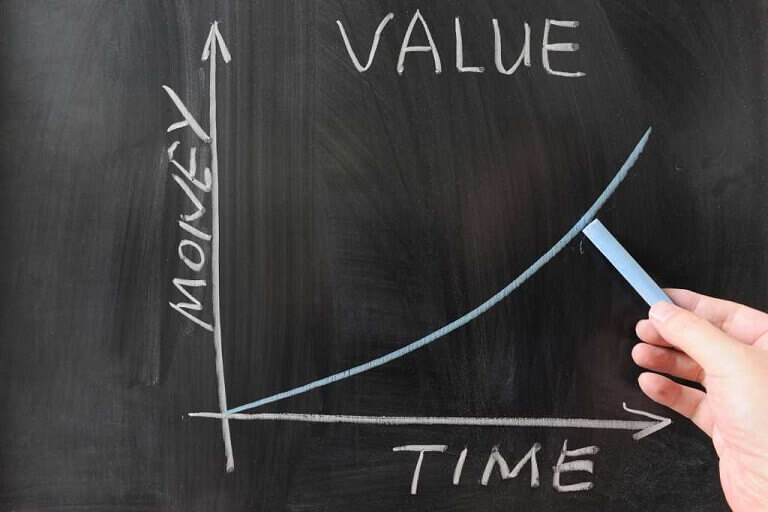# Time Value of Money - TVM

Updated on September 24, 2023 , 23647 views

## What is the Time Value of Money - TVM?

The time value of money (TVM) is the concept that money available at the present time is worth more than the identical sum in the future due to its potential earning capacity.This core principle of finance holds that, provided money can earn interest, any amount of money is worth more the sooner it is received. TVM is also sometimes referred to as present discounted value.

## Details of Time Value of Money - TVM

The time value of money draws from the idea that rational investors prefer to receive money today rather than the same amount of money in the future because of money's potential to grow in value over a given period of time. For example, money deposited into a Savings Account earns a certain interest rate, and is therefore said to be compounding in value.

Further illustrating the rational investor's preference, assume you have the option to choose between receiving Rs. 10,000 now versus Rs. 10,000 in two years. It's reasonable to assume most people would choose the first option. Despite the equal value at time of disbursement, receiving the Rs. 10,000 today has more value and utility to the beneficiary than receiving it in the future due to the opportunity costs associated with the wait. Such opportunity costs could include the potential gain on interest were that money received today and held in a savings account for two years.

Talk to our investment specialist
Disclaimer:
By submitting this form I authorize Fincash.com to call/SMS/email me about its products and I accept the terms of Privacy Policy and Terms & Conditions.

## Basic Time Value of Money Formula

Depending on the exact situation in question, the TVM formula may change slightly. For example, in the case of annuity or perpetuity payments, the generalized formula has additional or less factors. But in general, the most fundamental TVM formula takes into account the following variables:

• FV = Future value of money
• PV = present value of money
• i = interest rate
• n = number of compounding periods per year
• t = number of years

Based on these variables, the formula for TVM is:

FV = PV x [ 1 + (i / n) ] (n x t)

## Time Value of Money Example

Assume a sum of \$10,000 is invested for one year at 10% interest. The future value of that money is:

FV = Rs. 10,000 x (1 + (10% / 1) ^ (1 x 1) = Rs. 11,000

The formula can also be rearranged to find the value of the future sum in present day dollars. For example, the value of Rs. 5,000 one year from today, compounded at 7% interest, is:

PV = Rs. 5,000 / (1 + (7% / 1) ^ (1 x 1) = Rs. 4,673

## Effect of Compounding Periods on Future Value

The number of compounding periods can have a drastic effect on the TVM calculations. Taking the Rs. 10,000 example above, if the number of compounding periods is increased to quarterly, monthly or daily, the ending future value calculations are:

• Quarterly Compounding: FV = Rs 10,000 x (1 + (10% / 4) ^ (4 x 1) = `Rs. 11,038`
• Monthly Compounding: FV = Rs. 10,000 x (1 + (10% / 12) ^ (12 x 1) = `Rs. 11,047`
• Daily Compounding: FV = Rs. 10,000 x (1 + (10% / 365) ^ (365 x 1) = `Rs. 11,052`

This shows TVM depends not only on interest rate and time horizon, but also on how many times the compounding calculations are computed each year.

Disclaimer:
All efforts have been made to ensure the information provided here is accurate. However, no guarantees are made regarding correctness of data. Please verify with scheme information document before making any investment.